Filling up one area with a "oriented" polyline.

Recommended PostsHello guys,

Someone knows if's possible I fill up one area, as the picture, with one polyline oriented (or as a guided line), informing the lisp code the initial point, the radius of return line, and the space limits (as the limits line), horizontal or vertical to guide the lines until the whole area it's completely filled up?...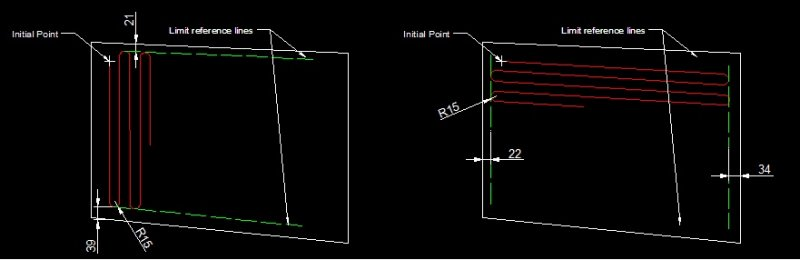Share on other sitesThis is my version, but I don't know what do you think - it may in some cases be shorter or longer fill than the opposite boundary edge (end of snake)...

```(defun c:snakefill (/ ptlst lxm uxm lym rym p1 p2 p3 p4 ip r d1 d2 d3 d4 dmin dir dx dy ls lr lx ly n ss)
(prompt "\nPick area...(lower-left corner+upper-right corner)")
(command "_.rectangle" pause pause)
(setq ptlst (mapcar 'cdr (vl-remove-if-not '(lambda (x) (eq (car x) 10)) (entget (entlast)))))
(setq ptlst (mapcar '(lambda (x) (trans x (entlast) 1)) ptlst))
(prompt "\nDelta X of OCS: ") (princ (distance (car ptlst) (cadr ptlst)))
(prompt "\nDelta Y of OCS: ") (princ (distance (cadr ptlst) (caddr ptlst)))
(initget 4)
(setq lxm (getdist "\nPick lower X of OCS offset margin <0.0>: "))
(if (null lxm) (setq lxm 0.0))
(initget 4)
(setq uxm (getdist "\nPick upper X of OCS offset margin <0.0>: "))
(if (null uxm) (setq uxm 0.0))
(initget 4)
(setq lym (getdist "\nPick left Y of OCS offset margin <0.0>: "))
(if (null lym) (setq lym 0.0))
(initget 4)
(setq rym (getdist "\nPick right Y of OCS offset margin <0.0>: "))
(if (null rym) (setq rym 0.0))
(setq p1 (mapcar '+ (car ptlst) (list lym lxm)))
(setq p2 (mapcar '+ (cadr ptlst) (list (- rym) lxm)))
(setq p3 (mapcar '+ (caddr ptlst) (list (- rym) (- uxm))))
(setq p4 (mapcar '+ (cadddr ptlst) (list lym (- uxm))))
(setq ip (getpoint "\nPick start point of snake fill: "))
(initget 7)
(setq r (getdist "\nPick radius of snake fill: "))
(setq d1 (distance ip p1) d2 (distance ip p2) d3 (distance ip p3) d4 (distance ip p4))
(setq dmin (min d1 d2 d3 d4))
(initget 1 "X Y")
(setq dir (getkword "\nSnake direction - parallel to X or Y of OCS (X/Y): "))
(if (equal dmin d1)
(progn
(setq dx (car (mapcar '- ip p1)))
(setq dy (cadr (mapcar '- ip p1)))
(if (eq dir "X")
(progn
(setq ls (- (distance p1 p2) dx r))
(setq lr (- (distance p1 p2) (* r 2.0)))
(setq ly (- (distance p1 p4) dy))
(setq n (fix (/ ly (* r 2.0))))
(command "_.pline" ip (polar ip 0.0 ls) "a" "s" (mapcar '+ (polar ip 0.0 ls) (list r r)) (mapcar '+ (polar ip 0.0 ls) (list 0.0 (* r 2.0))) "")
(command "_.pline" (mapcar '+ (polar ip 0.0 ls) (list 0.0 (* r 2.0))) (polar (mapcar '+ (polar ip 0.0 ls) (list 0.0 (* r 2.0))) pi lr) "a" "s" (mapcar '+ (polar (mapcar '+ (polar ip 0.0 ls) (list 0.0 (* r 2.0))) pi lr) (list (- r) r)) (mapcar '+ (polar (mapcar '+ (polar ip 0.0 ls) (list 0.0 (* r 2.0))) pi lr) (list 0.0 (* r 2.0))) "l" (polar (mapcar '+ (polar (mapcar '+ (polar ip 0.0 ls) (list 0.0 (* r 2.0))) pi lr) (list 0.0 (* r 2.0))) 0.0 lr) "a" "s" (mapcar '+ (polar (mapcar '+ (polar (mapcar '+ (polar ip 0.0 ls) (list 0.0 (* r 2.0))) pi lr) (list 0.0 (* r 2.0))) 0.0 lr) (list r r)) (mapcar '+ (polar (mapcar '+ (polar (mapcar '+ (polar ip 0.0 ls) (list 0.0 (* r 2.0))) pi lr) (list 0.0 (* r 2.0))) 0.0 lr) (list 0.0 (* r 2.0))) "")
(repeat (- (fix (/ n 2.0)) 1)
(command "_.copy" (entlast) "" '(0.0 0.0 0.0) (list 0.0 (* r 4.0) 0.0))
)
)
(progn
(setq ls (- (distance p1 p4) dy r))
(setq lr (- (distance p1 p4) (* r 2.0)))
(setq lx (- (distance p1 p2) dx))
(setq n (fix (/ lx (* r 2.0))))
(command "_.pline" ip (polar ip (* pi 0.5) ls) "a" "s" (mapcar '+ (polar ip (* pi 0.5) ls) (list r r)) (mapcar '+ (polar ip (* pi 0.5) ls) (list (* r 2.0) 0.0)) "")
(command "_.pline" (mapcar '+ (polar ip (* pi 0.5) ls) (list (* r 2.0) 0.0)) (polar (mapcar '+ (polar ip (* pi 0.5) ls) (list (* r 2.0) 0.0)) (* pi -0.5) lr) "a" "s" (mapcar '+ (polar (mapcar '+ (polar ip (* pi 0.5) ls) (list (* r 2.0) 0.0)) (* pi -0.5) lr) (list r (- r))) (mapcar '+ (polar (mapcar '+ (polar ip (* pi 0.5) ls) (list (* r 2.0) 0.0)) (* pi -0.5) lr) (list (* r 2.0) 0.0)) "l" (polar (mapcar '+ (polar (mapcar '+ (polar ip (* pi 0.5) ls) (list (* r 2.0) 0.0)) (* pi -0.5) lr) (list (* r 2.0) 0.0)) (* pi 0.5) lr) "a" "s" (mapcar '+ (polar (mapcar '+ (polar (mapcar '+ (polar ip (* pi 0.5) ls) (list (* r 2.0) 0.0)) (* pi -0.5) lr) (list (* r 2.0) 0.0)) (* pi 0.5) lr) (list r r)) (mapcar '+ (polar (mapcar '+ (polar (mapcar '+ (polar ip (* pi 0.5) ls) (list (* r 2.0) 0.0)) (* pi -0.5) lr) (list (* r 2.0) 0.0)) (* pi 0.5) lr) (list (* r 2.0) 0.0)) "")
(repeat (- (fix (/ n 2.0)) 1)
(command "_.copy" (entlast) "" '(0.0 0.0 0.0) (list (* r 4.0) 0.0 0.0))
)
)
)
)
)
(if (equal dmin d2)
(progn
(setq dx (car (mapcar '- p2 ip)))
(setq dy (cadr (mapcar '- ip p2)))
(if (eq dir "X")
(progn
(setq ls (- (distance p2 p1) dx r))
(setq lr (- (distance p2 p1) (* r 2.0)))
(setq ly (- (distance p2 p3) dy))
(setq n (fix (/ ly (* r 2.0))))
(command "_.pline" ip (polar ip pi ls) "a" "s" (mapcar '+ (polar ip pi ls) (list (- r) r)) (mapcar '+ (polar ip pi ls) (list 0.0 (* r 2.0))) "")
(command "_.pline" (mapcar '+ (polar ip pi ls) (list 0.0 (* r 2.0))) (polar (mapcar '+ (polar ip pi ls) (list 0.0 (* r 2.0))) 0.0 lr) "a" "s" (mapcar '+ (polar (mapcar '+ (polar ip pi ls) (list 0.0 (* r 2.0))) 0.0 lr) (list r r)) (mapcar '+ (polar (mapcar '+ (polar ip pi ls) (list 0.0 (* r 2.0))) 0.0 lr) (list 0.0 (* r 2.0))) "l" (polar (mapcar '+ (polar (mapcar '+ (polar ip pi ls) (list 0.0 (* r 2.0))) 0.0 lr) (list 0.0 (* r 2.0))) pi lr) "a" "s" (mapcar '+ (polar (mapcar '+ (polar (mapcar '+ (polar ip pi ls) (list 0.0 (* r 2.0))) 0.0 lr) (list 0.0 (* r 2.0))) pi lr) (list (- r) r)) (mapcar '+ (polar (mapcar '+ (polar (mapcar '+ (polar ip pi ls) (list 0.0 (* r 2.0))) 0.0 lr) (list 0.0 (* r 2.0))) pi lr) (list 0.0 (* r 2.0))) "")
(repeat (- (fix (/ n 2.0)) 1)
(command "_.copy" (entlast) "" '(0.0 0.0 0.0) (list 0.0 (* r 4.0) 0.0))
)
)
(progn
(setq ls (- (distance p2 p3) dy r))
(setq lr (- (distance p2 p3) (* r 2.0)))
(setq lx (- (distance p1 p2) dx))
(setq n (fix (/ lx (* r 2.0))))
(command "_.pline" ip (polar ip (* pi 0.5) ls) "a" "s" (mapcar '+ (polar ip (* pi 0.5) ls) (list (- r) r)) (mapcar '+ (polar ip (* pi 0.5) ls) (list (* r -2.0) 0.0)) "")
(command "_.pline" (mapcar '+ (polar ip (* pi 0.5) ls) (list (* r -2.0) 0.0)) (polar (mapcar '+ (polar ip (* pi 0.5) ls) (list (* r -2.0) 0.0)) (* pi -0.5) lr) "a" "s" (mapcar '+ (polar (mapcar '+ (polar ip (* pi 0.5) ls) (list (* r -2.0) 0.0)) (* pi -0.5) lr) (list (- r) (- r))) (mapcar '+ (polar (mapcar '+ (polar ip (* pi 0.5) ls) (list (* r -2.0) 0.0)) (* pi -0.5) lr) (list (* r -2.0) 0.0)) "l" (polar (mapcar '+ (polar (mapcar '+ (polar ip (* pi 0.5) ls) (list (* r -2.0) 0.0)) (* pi -0.5) lr) (list (* r -2.0) 0.0)) (* pi 0.5) lr) "a" "s" (mapcar '+ (polar (mapcar '+ (polar (mapcar '+ (polar ip (* pi 0.5) ls) (list (* r -2.0) 0.0)) (* pi -0.5) lr) (list (* r -2.0) 0.0)) (* pi 0.5) lr) (list (- r) r)) (mapcar '+ (polar (mapcar '+ (polar (mapcar '+ (polar ip (* pi 0.5) ls) (list (* r -2.0) 0.0)) (* pi -0.5) lr) (list (* r -2.0) 0.0)) (* pi 0.5) lr) (list (* r -2.0) 0.0)) "")
(repeat (- (fix (/ n 2.0)) 1)
(command "_.copy" (entlast) "" '(0.0 0.0 0.0) (list (* r -4.0) 0.0 0.0))
)
)
)
)
)
(if (equal dmin d3)
(progn
(setq dx (car (mapcar '- p3 ip)))
(setq dy (cadr (mapcar '- p3 ip)))
(if (eq dir "X")
(progn
(setq ls (- (distance p3 p4) dx r))
(setq lr (- (distance p3 p4) (* r 2.0)))
(setq ly (- (distance p3 p2) dy))
(setq n (fix (/ ly (* r 2.0))))
(command "_.pline" ip (polar ip pi ls) "a" "s" (mapcar '+ (polar ip pi ls) (list (- r) (- r))) (mapcar '+ (polar ip pi ls) (list 0.0 (* r -2.0))) "")
(command "_.pline" (mapcar '+ (polar ip pi ls) (list 0.0 (* r -2.0))) (polar (mapcar '+ (polar ip pi ls) (list 0.0 (* r -2.0))) 0.0 lr) "a" "s" (mapcar '+ (polar (mapcar '+ (polar ip pi ls) (list 0.0 (* r -2.0))) 0.0 lr) (list r (- r))) (mapcar '+ (polar (mapcar '+ (polar ip pi ls) (list 0.0 (* r -2.0))) 0.0 lr) (list 0.0 (* r -2.0))) "l" (polar (mapcar '+ (polar (mapcar '+ (polar ip pi ls) (list 0.0 (* r -2.0))) 0.0 lr) (list 0.0 (* r -2.0))) pi lr) "a" "s" (mapcar '+ (polar (mapcar '+ (polar (mapcar '+ (polar ip pi ls) (list 0.0 (* r -2.0))) 0.0 lr) (list 0.0 (* r -2.0))) pi lr) (list (- r) (- r))) (mapcar '+ (polar (mapcar '+ (polar (mapcar '+ (polar ip pi ls) (list 0.0 (* r -2.0))) 0.0 lr) (list 0.0 (* r -2.0))) pi lr) (list 0.0 (* r -2.0))) "")
(repeat (- (fix (/ n 2.0)) 1)
(command "_.copy" (entlast) "" '(0.0 0.0 0.0) (list 0.0 (* r -4.0) 0.0))
)
)
(progn
(setq ls (- (distance p3 p2) dy r))
(setq lr (- (distance p3 p2) (* r 2.0)))
(setq lx (- (distance p3 p4) dx))
(setq n (fix (/ lx (* r 2.0))))
(command "_.pline" ip (polar ip (* pi -0.5) ls) "a" "s" (mapcar '+ (polar ip (* pi -0.5) ls) (list (- r) (- r))) (mapcar '+ (polar ip (* pi -0.5) ls) (list (* r -2.0) 0.0)) "")
(command "_.pline" (mapcar '+ (polar ip (* pi -0.5) ls) (list (* r -2.0) 0.0)) (polar (mapcar '+ (polar ip (* pi -0.5) ls) (list (* r -2.0) 0.0)) (* pi 0.5) lr) "a" "s" (mapcar '+ (polar (mapcar '+ (polar ip (* pi -0.5) ls) (list (* r -2.0) 0.0)) (* pi 0.5) lr) (list (- r) r)) (mapcar '+ (polar (mapcar '+ (polar ip (* pi -0.5) ls) (list (* r -2.0) 0.0)) (* pi 0.5) lr) (list (* r -2.0) 0.0)) "l" (polar (mapcar '+ (polar (mapcar '+ (polar ip (* pi -0.5) ls) (list (* r -2.0) 0.0)) (* pi 0.5) lr) (list (* r -2.0) 0.0)) (* pi -0.5) lr) "a" "s" (mapcar '+ (polar (mapcar '+ (polar (mapcar '+ (polar ip (* pi -0.5) ls) (list (* r -2.0) 0.0)) (* pi 0.5) lr) (list (* r -2.0) 0.0)) (* pi -0.5) lr) (list (- r) (- r))) (mapcar '+ (polar (mapcar '+ (polar (mapcar '+ (polar ip (* pi -0.5) ls) (list (* r -2.0) 0.0)) (* pi 0.5) lr) (list (* r -2.0) 0.0)) (* pi -0.5) lr) (list (* r -2.0) 0.0)) "")
(repeat (- (fix (/ n 2.0)) 1)
(command "_.copy" (entlast) "" '(0.0 0.0 0.0) (list (* r -4.0) 0.0 0.0))
)
)
)
)
)
(if (equal dmin d4)
(progn
(setq dx (car (mapcar '- ip p4)))
(setq dy (cadr (mapcar '- p4 ip)))
(if (eq dir "X")
(progn
(setq ls (- (distance p4 p3) dx r))
(setq lr (- (distance p4 p3) (* r 2.0)))
(setq ly (- (distance p4 p1) dy))
(setq n (fix (/ ly (* r 2.0))))
(command "_.pline" ip (polar ip 0.0 ls) "a" "s" (mapcar '+ (polar ip 0.0 ls) (list r (- r))) (mapcar '+ (polar ip 0.0 ls) (list 0.0 (* r -2.0))) "")
(command "_.pline" (mapcar '+ (polar ip 0.0 ls) (list 0.0 (* r -2.0))) (polar (mapcar '+ (polar ip 0.0 ls) (list 0.0 (* r -2.0))) pi lr) "a" "s" (mapcar '+ (polar (mapcar '+ (polar ip 0.0 ls) (list 0.0 (* r -2.0))) pi lr) (list (- r) (- r))) (mapcar '+ (polar (mapcar '+ (polar ip 0.0 ls) (list 0.0 (* r -2.0))) pi lr) (list 0.0 (* r -2.0))) "l" (polar (mapcar '+ (polar (mapcar '+ (polar ip 0.0 ls) (list 0.0 (* r -2.0))) pi lr) (list 0.0 (* r -2.0))) 0.0 lr) "a" "s" (mapcar '+ (polar (mapcar '+ (polar (mapcar '+ (polar ip 0.0 ls) (list 0.0 (* r -2.0))) pi lr) (list 0.0 (* r -2.0))) 0.0 lr) (list r (- r))) (mapcar '+ (polar (mapcar '+ (polar (mapcar '+ (polar ip 0.0 ls) (list 0.0 (* r -2.0))) pi lr) (list 0.0 (* r -2.0))) 0.0 lr) (list 0.0 (* r -2.0))) "")
(repeat (- (fix (/ n 2.0)) 1)
(command "_.copy" (entlast) "" '(0.0 0.0 0.0) (list 0.0 (* r -4.0) 0.0))
)
)
(progn
(setq ls (- (distance p4 p1) dy r))
(setq lr (- (distance p4 p1) (* r 2.0)))
(setq lx (- (distance p4 p3) dx))
(setq n (fix (/ lx (* r 2.0))))
(command "_.pline" ip (polar ip (* pi -0.5) ls) "a" "s" (mapcar '+ (polar ip (* pi -0.5) ls) (list r (- r))) (mapcar '+ (polar ip (* pi -0.5) ls) (list (* r 2.0) 0.0)) "")
(command "_.pline" (mapcar '+ (polar ip (* pi -0.5) ls) (list (* r 2.0) 0.0)) (polar (mapcar '+ (polar ip (* pi -0.5) ls) (list (* r 2.0) 0.0)) (* pi 0.5) lr) "a" "s" (mapcar '+ (polar (mapcar '+ (polar ip (* pi -0.5) ls) (list (* r 2.0) 0.0)) (* pi 0.5) lr) (list r r)) (mapcar '+ (polar (mapcar '+ (polar ip (* pi -0.5) ls) (list (* r 2.0) 0.0)) (* pi 0.5) lr) (list (* r 2.0) 0.0)) "l" (polar (mapcar '+ (polar (mapcar '+ (polar ip (* pi -0.5) ls) (list (* r 2.0) 0.0)) (* pi 0.5) lr) (list (* r 2.0) 0.0)) (* pi -0.5) lr) "a" "s" (mapcar '+ (polar (mapcar '+ (polar (mapcar '+ (polar ip (* pi -0.5) ls) (list (* r 2.0) 0.0)) (* pi 0.5) lr) (list (* r 2.0) 0.0)) (* pi -0.5) lr) (list r (- r))) (mapcar '+ (polar (mapcar '+ (polar (mapcar '+ (polar ip (* pi -0.5) ls) (list (* r 2.0) 0.0)) (* pi 0.5) lr) (list (* r 2.0) 0.0)) (* pi -0.5) lr) (list (* r 2.0) 0.0)) "")
(repeat (- (fix (/ n 2.0)) 1)
(command "_.copy" (entlast) "" '(0.0 0.0 0.0) (list (* r 4.0) 0.0 0.0))
)
)
)
)
)
(command "_.pedit" "m" ss "" "j" "" "")
(princ)
)
```

M.R.

Edited by marko_ribar

Share on other sitesThis is close also lines can be any angel etc done for chevrons in road islands just create an inner polygon

```; this use the extrim command to trim shape
; By Alan H Jan 2012
(defun C:Chevron ( / obj pt1 pt2 pt3 pt4 newpt1 newpt2 )
(acet-error-init (list
(list   "cmdecho" 0
"highlight" 0
"regenmode" 1
"osmode" 0
"ucsicon" 0
"offsetdist" 0
"attreq" 0
"plinewid" 0
"plinetype" 1
"gridmode" 0
"celtype" "CONTINUOUS"
"ucsfollow" 0
"limcheck" 0
)
T     ;flag. True means use undo for error clean up.
'(if redraw_it (redraw na 4))
);list
);acet-error-init
(setq obj (car (entsel "\nPick pline or circle")))
; should do a object test here
(setq whatis (cdr (assoc 0 (entget obj))))
(if (= whatis "LWPOLYLINE")
(princ)
(progn
(princ "\You have picked something other than a polyline ")
(princ "\Remake into a pline and do again ")
(setq dummy (getstring "\press any key"))
(exit)
) ; progn
) ; if
(setq pt1 (Getpoint "\nPick Line start point"))
(setq pt2 (Getpoint pt1 "\nPick end point"))
(command "line" pt1 pt2 "")
(setq gap1 (getreal "\nenter spacing 1"))
(setq gap2 (getreal "\nenter spacing 2"))
(setq pt3 (getpoint "\nPick 1st cross point"))
(setq pt4 (getpoint pt3 "\nPick 2nd cross point"))
(setq dist (distance pt3 pt4))
(setq x (fix (/ dist (+ gap1 gap2))))
(setq newpt1 (strcat (rtos gap1 2 2) ",0.0"))
(setq newpt2 (strcat (rtos gap2 2 2) ",0.0"))
(repeat x
(command "copy" "L" "" "0,0" newpt1)
(command "copy" "L" "" "0,0" newpt2)
)
(etrim obj pt1)
(acet-error-restore)
) ; end defun

(princ)```

Share on other sitesThis is my version, but I don't know what do you think - it may in some cases be shorter or longer fill than the opposite boundary edge (end of snake)...

M.R.

Marko_ribar, thanks for help me, the code that you wrote it's almost that I need, but the lines are drawed only in a regular region (rectangle)? It's possible select other kind of region? And as the picture, the arcs following the limits of inclination... I was thinking in some thing like I fill up the region following the limits using as a "rule" to drawing the line, like: if the start point was inserted it's possible to draw the line until the limit line and where we get crossing point of the limit line (green line) it could be decreased to creat the end of first line using the radius as a reference, after that drawing the arc and follow again the "rule", doing it until the end of drawing, but I don't know if's possible work in this way.

Thank's again and sorry about the english!...

Share on other sitesThis is close also lines can be any angel etc done for chevrons in road islands just create an inner polygon

BIGAL, thanks, I don't know if I'm doing it wrong, but using your code I create only lines following the region (outline), is it correct?...

Share on other sitesYes only uses 1 pline but it can have arcs etc

Share on other sitesThis request reminds me of this thread - perhaps that program may help.

Share on other sitesThis request reminds me of this thread - perhaps that program may help.

Lee Mac is almost that... but, I'm filling up a irregular region!... I'm showing another drawing to explain about it...

Share on other sitesIt is solvable but its not a quick task, so I am only offering maybe a method Lee may be able to help with code

offset outside pline as required

create line at required angle and spacing (see my chevron.lsp) trim to new inner pline

using a list of the lines left to right just alternate the fillet command top and bottom

should look like image.

Share on other sitesThanks Bigal...

Share on other sitesCould you help me with this code?...

Share on other sitesCafeJr, here try this and reply if something's wrong... It should now work with 2 opposite open 2d curve boundaries...

```(defun c:snakefill-2curveboundaries (/ *adoc* odd even osm pea ch r lpl upl ip dir lplo uplo spc xl1 c1 xl2 c2 cl ss)

(defun odd (lst)
(if lst (cons (car lst) (odd (cddr lst))))
)

(defun even (lst)
(if lst (cons (cadr lst) (even (cddr lst))))
)

(setq osm (getvar 'osmode))
(setq pea (getvar 'peditaccept))
(setvar 'osmode 0)
(command "_.ucs" "w")
(initget 1 "Left-Right Up-Down")
(setq ch (getkword "\nChoose option (Left-Right / Up-Down) curve boundaries: "))
(initget 7)
(setq r (getdist "\nSpecify radius of snake turn: "))
(if (eq ch "Up-Down")
(progn
(setq lpl (car (entsel "\nPick lower curve boundary...")))
(setq upl (car (entsel "\nPick upper curve boundary...")))
(setq ip (getpoint "\nPick start point (\"left\" - Up-Down or \"bottom\" - Left-Right): "))
(initget 1 "Up Down")
(setq dir (getkword "\nChoose start direction (Up / Down): "))
(if (eq dir "Up")
(progn
(command "_.offset" r lpl ip "")
(setq lplo (entlast))
(command "_.offset" r upl ip "")
(setq uplo (entlast))
(setq spc (vla-ObjectIdToObject *adoc* (vla-get-OwnerId (vlax-ename->vla-object lpl))))
(setq c1 T c2 T k -3.0)
(while (and c1 c2)
(setq xl1 (vlax-invoke spc 'addxline (polar ip 0.0 (* r (setq k (+ k 4.0)))) (polar (polar ip 0.0 (* r k)) (* pi 0.5) 1.0)))
(setq c1 (vlax-invoke xl1 'intersectwith (vlax-ename->vla-object uplo) acextendnone))
(vla-delete xl1)
(setq xl2 (vlax-invoke spc 'addxline (polar ip 0.0 (* r (+ k 2.0))) (polar (polar ip 0.0 (* r (+ k 2.0))) (* pi 0.5) 1.0)))
(setq c2 (vlax-invoke xl2 'intersectwith (vlax-ename->vla-object lplo) acextendnone))
(vla-delete xl2)
(if c1 (setq cl (cons c1 cl)))
(if (and c1 c2) (setq cl (cons c2 cl)))
)
(setq cl (reverse cl))
(foreach c (vl-remove nil (odd cl))
(command "_.arc" (polar c pi r) (polar c (* pi 0.5) r) (polar c 0.0 r))
)
(foreach c (vl-remove nil (even cl))
(command "_.arc" (polar c pi r) (polar c (* pi -0.5) r) (polar c 0.0 r))
)
(mapcar '(lambda (p1 p2) (progn (command "_.line" p1 p2 "") (ssadd (entlast) ss))) (mapcar '(lambda (p) (polar p 0.0 r)) (vl-remove nil (odd cl))) (mapcar '(lambda (p) (polar p pi r)) (vl-remove nil (even cl))))
(mapcar '(lambda (p1 p2) (progn (command "_.line" p1 p2 "") (ssadd (entlast) ss))) (mapcar '(lambda (p) (polar p 0.0 r)) (vl-remove nil (even cl))) (mapcar '(lambda (p) (polar p pi r)) (vl-remove nil (cdr (odd cl)))))
(command "_.line" ip (polar (car cl) pi r) "")
)
(progn
(command "_.offset" r lpl ip "")
(setq lplo (entlast))
(command "_.offset" r upl ip "")
(setq uplo (entlast))
(setq spc (vla-ObjectIdToObject *adoc* (vla-get-OwnerId (vlax-ename->vla-object lpl))))
(setq c1 T c2 T k -3.0)
(while (and c1 c2)
(setq xl1 (vlax-invoke spc 'addxline (polar ip 0.0 (* r (setq k (+ k 4.0)))) (polar (polar ip 0.0 (* r k)) (* pi 0.5) 1.0)))
(setq c1 (vlax-invoke xl1 'intersectwith (vlax-ename->vla-object lplo) acextendnone))
(vla-delete xl1)
(setq xl2 (vlax-invoke spc 'addxline (polar ip 0.0 (* r (+ k 2.0))) (polar (polar ip 0.0 (* r (+ k 2.0))) (* pi 0.5) 1.0)))
(setq c2 (vlax-invoke xl2 'intersectwith (vlax-ename->vla-object uplo) acextendnone))
(vla-delete xl2)
(if c1 (setq cl (cons c1 cl)))
(if (and c1 c2) (setq cl (cons c2 cl)))
)
(setq cl (reverse cl))
(foreach c (vl-remove nil (odd cl))
(command "_.arc" (polar c pi r) (polar c (* pi -0.5) r) (polar c 0.0 r))
)
(foreach c (vl-remove nil (even cl))
(command "_.arc" (polar c pi r) (polar c (* pi 0.5) r) (polar c 0.0 r))
)
(mapcar '(lambda (p1 p2) (progn (command "_.line" p1 p2 "") (ssadd (entlast) ss))) (mapcar '(lambda (p) (polar p 0.0 r)) (vl-remove nil (odd cl))) (mapcar '(lambda (p) (polar p pi r)) (vl-remove nil (even cl))))
(mapcar '(lambda (p1 p2) (progn (command "_.line" p1 p2 "") (ssadd (entlast) ss))) (mapcar '(lambda (p) (polar p 0.0 r)) (vl-remove nil (even cl))) (mapcar '(lambda (p) (polar p pi r)) (vl-remove nil (cdr (odd cl)))))
(command "_.line" ip (polar (car cl) pi r) "")
)
)
)
(progn
(setq lpl (car (entsel "\nPick right curve boundary...")))
(setq upl (car (entsel "\nPick left curve boundary...")))
(setq ip (getpoint "\nPick start point (\"left\" - Up-Down or \"bottom\" - Left-Right): "))
(initget 1 "Left Right")
(setq dir (getkword "\nChoose start direction (Left / Right): "))
(if (eq dir "Left")
(progn
(command "_.offset" r lpl ip "")
(setq lplo (entlast))
(command "_.offset" r upl ip "")
(setq uplo (entlast))
(setq spc (vla-ObjectIdToObject *adoc* (vla-get-OwnerId (vlax-ename->vla-object lpl))))
(setq c1 T c2 T k -3.0)
(while (and c1 c2)
(setq xl1 (vlax-invoke spc 'addxline (polar ip (* pi 0.5) (* r (setq k (+ k 4.0)))) (polar (polar ip (* pi 0.5) (* r k)) 0.0 1.0)))
(setq c1 (vlax-invoke xl1 'intersectwith (vlax-ename->vla-object uplo) acextendnone))
(vla-delete xl1)
(setq xl2 (vlax-invoke spc 'addxline (polar ip (* pi 0.5) (* r (+ k 2.0))) (polar (polar ip (* pi 0.5) (* r (+ k 2.0))) 0.0 1.0)))
(setq c2 (vlax-invoke xl2 'intersectwith (vlax-ename->vla-object lplo) acextendnone))
(vla-delete xl2)
(if c1 (setq cl (cons c1 cl)))
(if (and c1 c2) (setq cl (cons c2 cl)))
)
(setq cl (reverse cl))
(foreach c (vl-remove nil (odd cl))
(command "_.arc" (polar c (* pi -0.5) r) (polar c pi r) (polar c (* pi 0.5) r))
)
(foreach c (vl-remove nil (even cl))
(command "_.arc" (polar c (* pi -0.5) r) (polar c 0.0 r) (polar c (* pi 0.5) r))
)
(mapcar '(lambda (p1 p2) (progn (command "_.line" p1 p2 "") (ssadd (entlast) ss))) (mapcar '(lambda (p) (polar p (* pi 0.5) r)) (vl-remove nil (odd cl))) (mapcar '(lambda (p) (polar p (* pi -0.5) r)) (vl-remove nil (even cl))))
(mapcar '(lambda (p1 p2) (progn (command "_.line" p1 p2 "") (ssadd (entlast) ss))) (mapcar '(lambda (p) (polar p (* pi 0.5) r)) (vl-remove nil (even cl))) (mapcar '(lambda (p) (polar p (* pi -0.5) r)) (vl-remove nil (cdr (odd cl)))))
(command "_.line" ip (polar (car cl) (* pi -0.5) r) "")
)
(progn
(command "_.offset" r lpl ip "")
(setq lplo (entlast))
(command "_.offset" r upl ip "")
(setq uplo (entlast))
(setq spc (vla-ObjectIdToObject *adoc* (vla-get-OwnerId (vlax-ename->vla-object lpl))))
(setq c1 T c2 T k -3.0)
(while (and c1 c2)
(setq xl1 (vlax-invoke spc 'addxline (polar ip (* pi 0.5) (* r (setq k (+ k 4.0)))) (polar (polar ip (* pi 0.5) (* r k)) 0.0 1.0)))
(setq c1 (vlax-invoke xl1 'intersectwith (vlax-ename->vla-object lplo) acextendnone))
(vla-delete xl1)
(setq xl2 (vlax-invoke spc 'addxline (polar ip (* pi 0.5) (* r (+ k 2.0))) (polar (polar ip (* pi 0.5) (* r (+ k 2.0))) 0.0 1.0)))
(setq c2 (vlax-invoke xl2 'intersectwith (vlax-ename->vla-object uplo) acextendnone))
(vla-delete xl2)
(if c1 (setq cl (cons c1 cl)))
(if (and c1 c2) (setq cl (cons c2 cl)))
)
(setq cl (reverse cl))
(foreach c (vl-remove nil (odd cl))
(command "_.arc" (polar c (* pi -0.5) r) (polar c 0.0 r) (polar c (* pi 0.5) r))
)
(foreach c (vl-remove nil (even cl))
(command "_.arc" (polar c (* pi -0.5) r) (polar c pi r) (polar c (* pi 0.5) r))
)
(mapcar '(lambda (p1 p2) (progn (command "_.line" p1 p2 "") (ssadd (entlast) ss))) (mapcar '(lambda (p) (polar p (* pi 0.5) r)) (vl-remove nil (odd cl))) (mapcar '(lambda (p) (polar p (* pi -0.5) r)) (vl-remove nil (even cl))))
(mapcar '(lambda (p1 p2) (progn (command "_.line" p1 p2 "") (ssadd (entlast) ss))) (mapcar '(lambda (p) (polar p (* pi 0.5) r)) (vl-remove nil (even cl))) (mapcar '(lambda (p) (polar p (* pi -0.5) r)) (vl-remove nil (cdr (odd cl)))))
(command "_.line" ip (polar (car cl) (* pi -0.5) r) "")
)
)
)
)
(setvar 'peditaccept 1)
(command "_.pedit" "m" ss "" "j" "" "")
(entdel lplo)
(entdel uplo)
(setvar 'osmode osm)
(setvar 'peditaccept pea)
(command "_.ucs" "p")
(princ)
)

(defun c:sf-2c nil (c:snakefill-2curveboundaries))
(prompt "\n...Run with 'SF-2C'...")
(princ)
```

M.R.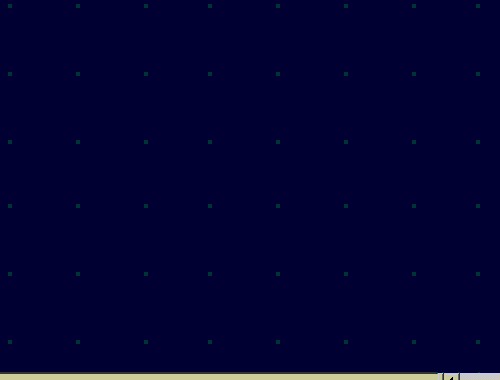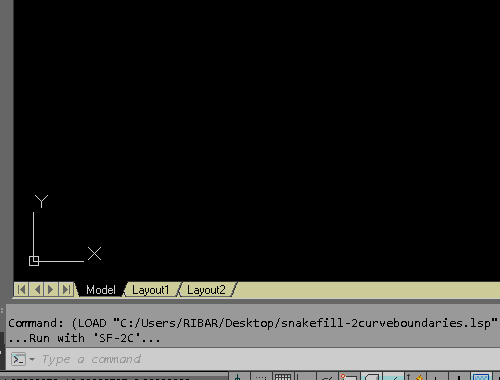Edited by marko_ribar

Share on other sitesMarko_ribar Very very nice new it was solvable

Share on other sites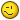Thanks, BIGAL... I'll attach more complete version - it determines where picked point is and according to that it proceeds to draw snake to the opposite side of area between curves...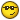M.R.

snakefill-2curveboundaries.lsp

Edited by marko_ribar

Share on other sites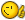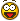Thanks, BIGAL... I'll attach more complete version - it determines where picked point is and according to that it proceeds to draw snake to the opposite side of area between curves...M.R.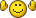Wowwwwwwwwwwwwww... Thank you a lot Marko_ribar!!!... It's exactly that I need!!!... I'm gratefully!!!... It's working as good as I need!...

Share on other sitesThanks, BIGAL... I'll attach more complete version - it determines where picked point is and according to that it proceeds to draw snake to the opposite side of area between curves...M.R.

Master Marko_ribar,

I don't know if is it another thread, but, I was thinking that this code would be one "esay" code, I'm really shocked about it! It was a big code and NOT so easy!!!... Well, to this code follow one reference line of the boundary plan like the picture, is it possible?

Thank you a lot!!!... I'm really grateful!!!...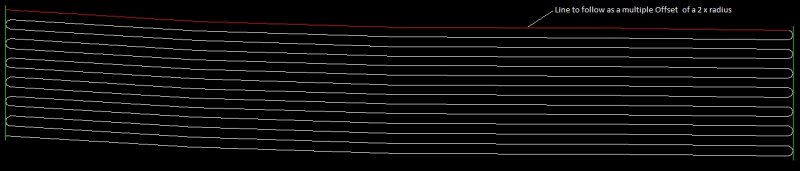Share on other sitesIf 3rd boundary is an arc I think it's possible... But bend must be between 1st and 2nd side boundaries - something like circle isn't possible... And I think this is an example of multiple copy of 3rd boundary in vertical Up/Down direction rather than offsetting... As I said if it's arc than it's possible... The princip is the same as above posted code... Just instead of Xlines you need intersect with Arcs in Horizontal/Vertical directions... I think you have much info to construct this on your own... If you want you can show us your result... We'll be happy to see that it works... Just keep in mind when searching for centers of side arcs I'd use 'intersectwith method with option acextendthisentity (arc is the first VLA-OBJECT)...

M.R.

Share on other sitesIf 3rd boundary is an arc I think it's possible... But bend must be between 1st and 2nd side boundaries - something like circle isn't possible... And I think this is an example of multiple copy of 3rd boundary in vertical Up/Down direction rather than offsetting... As I said if it's arc than it's possible... The princip is the same as above posted code... Just instead of Xlines you need intersect with Arcs in Horizontal/Vertical directions... I think you have much info to construct this on your own... If you want you can show us your result... We'll be happy to see that it works... Just keep in mind when searching for centers of side arcs I'd use 'intersectwith method with option acextendthisentity (arc is the first VLA-OBJECT)...

M.R.

In fact the boundary line is a pline limit of one area that I has to fill up, It's like the next picture... I'm trying to fill it with a "snake line" following some rules. I can say you that it's only the first step of the drawing! But is the worst of them...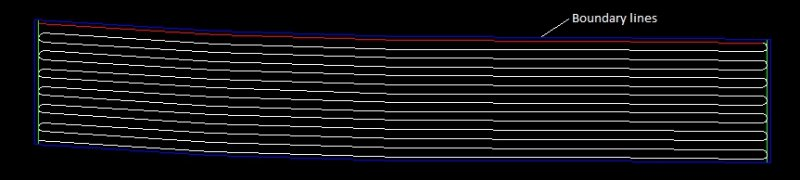EDIT: Opsss... Sorry, but, are you ask me to show you the result of it?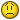I have no ideia how to do in this way, I'm studying another way to do, but trying to compile another codes!...

Edited by CafeJr

Share on other sitesHere is the lisp... It works with arcs as third boundary... 1st and 2nd side boundaries must be horizontal or vertical curves...

I didn't use acextendthisentity, but had to recreate half of circle arc...

M.R.

[EDIT : I don't know why, but it doesn't work on A2012 and it works on A2014]...

[EDIT2 : It now works and on versions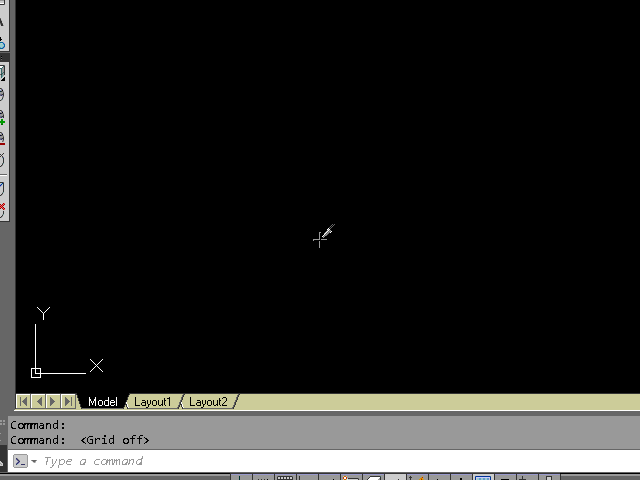snakefill-2lineboundaries-arc - entmake arcs.lsp

Edited by marko_ribar
EDIT note

Share on other sitesHere is the lisp... It works with arcs as third boundary... 1st and 2nd side boundaries must be horizontal or vertical lines...

I didn't use acextendthisentity, but had to recreate half of circle arc...

M.R.

[EDIT : I don't know why, but it doesn't work on A2012 and it works on A2014]...

Marco_ribar, thank you a lot to help me!!!... It's almost that, on the last two pictures you can see that the line to be followed is the boundary line (in that case a horizontal line), instead of draw a arc, it's possible to select that line as a reference line? After that do the offsets from up side to down closing with arcs... I'm trying to do some thing compiling codes, like a offsets off the line and arcs on that situation, after doing a "pedit" to join these objects...

Edited by CafeJr

Join the conversation

You can post now and register later. If you have an account, sign in now to post with your account.
Note: Your post will require moderator approval before it will be visible.×   Pasted as rich text.   Paste as plain text instead

Only 75 emoji are allowed.